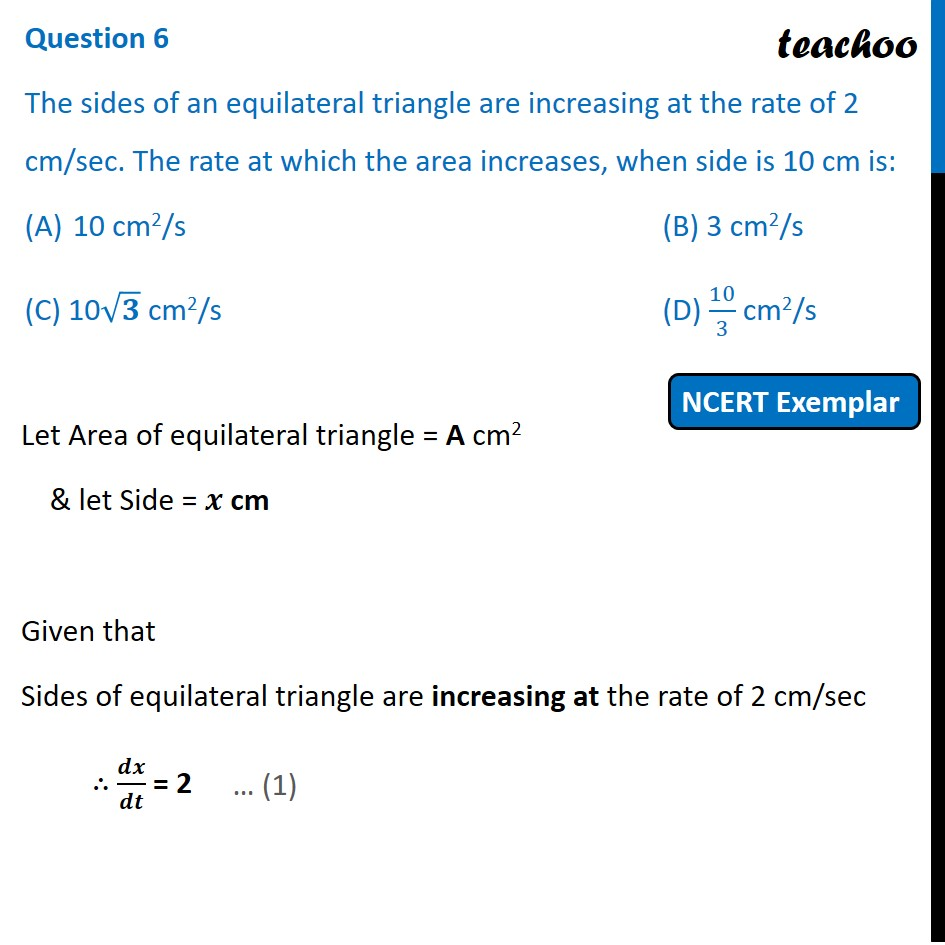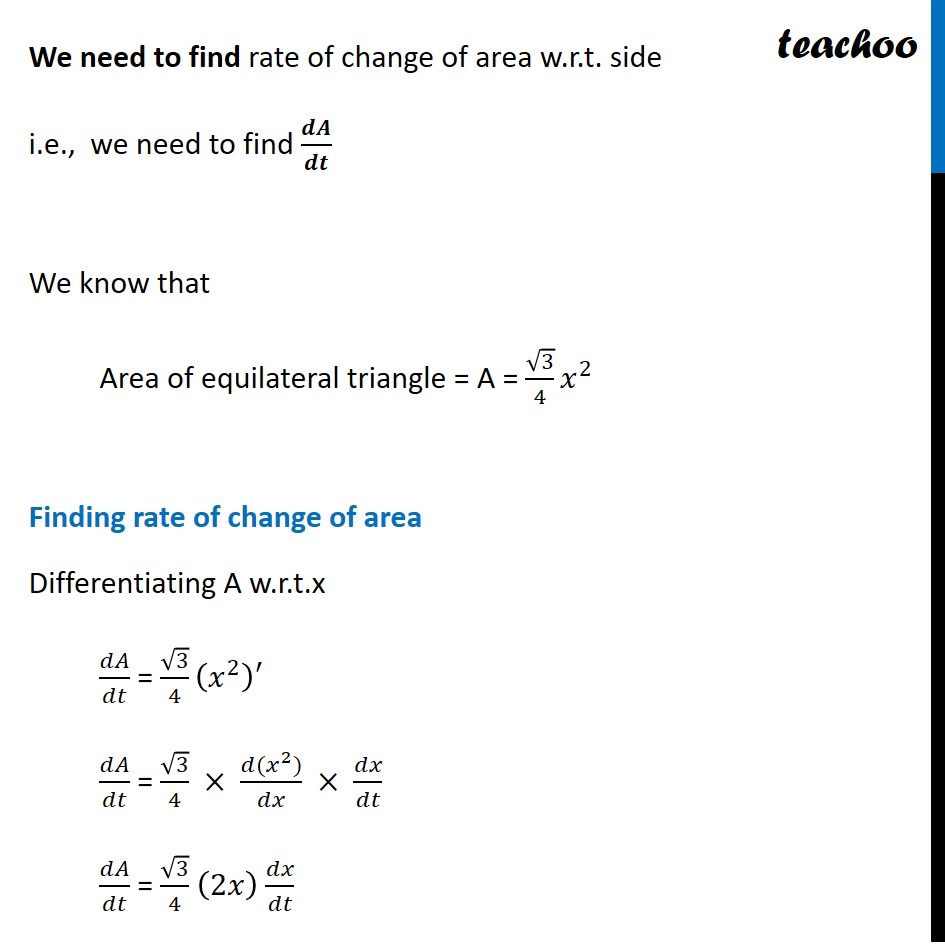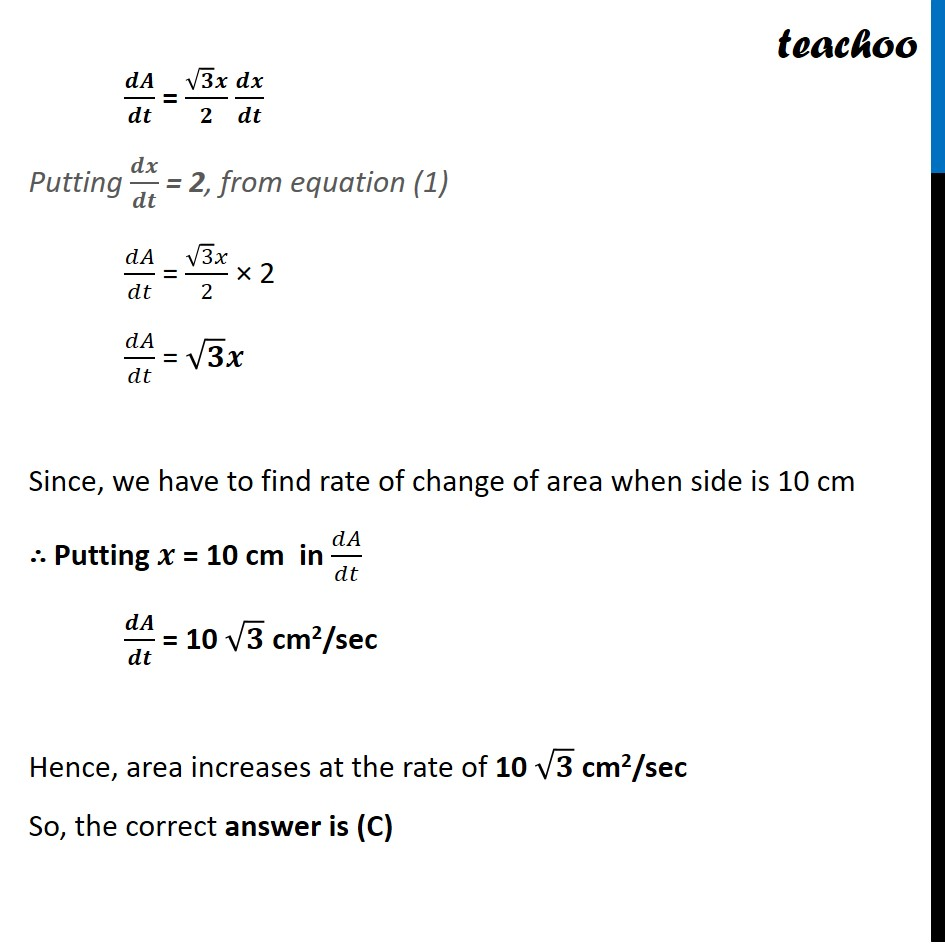NCERT Exemplar - MCQs

Chapter 6 Class 12 Application of Derivatives
Serial order wise

## (C) 10 √ 3 cm 2 /s               (D) 10/3 cm 2 /s

This question is similar to Ex 6.1, 1 - Chapter 6 Class 12 - Application of DerivativesLearn in your speed, with individual attention - Teachoo Maths 1-on-1 Class

### Transcript

Question 1 The sides of an equilateral triangle are increasing at the rate of 2 cm/sec. The rate at which the area increases, when side is 10 cm is: 10 cm2/s (B) 3 cm2/s (C) 10√𝟑 cm2/s (D) 10/3 cm2/s Let Area of equilateral triangle = A cm2 & let Side = 𝒙 cm Given that Sides of equilateral triangle are increasing at the rate of 2 cm/sec ∴ 𝒅𝒙/𝒅𝒕 = 2 We need to find rate of change of area w.r.t. side i.e., we need to find 𝒅𝑨/𝒅𝒕 We know that Area of equilateral triangle = A = √3/4 𝑥^2 Finding rate of change of area Differentiating A w.r.t.x 𝑑𝐴/𝑑𝑡 = √3/4 (𝑥^2 )′ 𝑑𝐴/𝑑𝑡 = √3/4 × (𝑑〖(𝑥〗^2))/𝑑𝑥 × 𝑑𝑥/𝑑𝑡 𝑑𝐴/𝑑𝑡 = √3/4 (2𝑥) 𝑑𝑥/𝑑𝑡 𝒅𝑨/𝒅𝒕 = (√𝟑 𝒙)/𝟐 𝒅𝒙/𝒅𝒕 Putting 𝒅𝒙/𝒅𝒕 = 2, from equation (1) 𝑑𝐴/𝑑𝑡 = (√3 𝑥)/2 × 2 𝑑𝐴/𝑑𝑡 = √𝟑 𝒙 Since, we have to find rate of change of area when side is 10 cm ∴ Putting 𝒙 = 10 cm in 𝑑𝐴/𝑑𝑡 𝒅𝑨/𝒅𝒕 = 10 √𝟑 cm2/sec Hence, area increases at the rate of 10 √𝟑 cm2/sec So, the correct answer is (C) 𝒅𝑨/𝒅𝒕 = (√𝟑 𝒙)/𝟐 𝒅𝒙/𝒅𝒕 Putting 𝒅𝒙/𝒅𝒕 = 2, from equation (1) 𝑑𝐴/𝑑𝑡 = (√3 𝑥)/2 × 2 𝑑𝐴/𝑑𝑡 = √𝟑 𝒙 Since, we have to find rate of change of area when side is 10 cm ∴ Putting 𝒙 = 10 cm in 𝑑𝐴/𝑑𝑡 𝒅𝑨/𝒅𝒕 = 10 √𝟑 cm2/sec Hence, area increases at the rate of 10 √𝟑 cm2/sec So, the correct answer is (C)